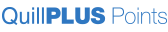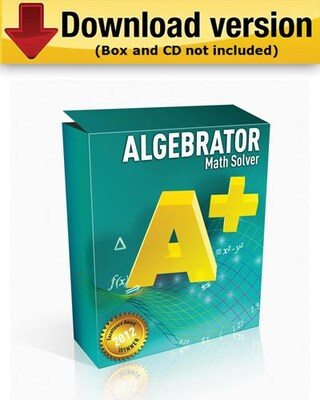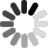A small part of your job is 100% of ours.Limited Time- join for only \$1.99 and receive 500 Bonus QPoints

# Algebrator for Windows (1 - User) [Download]

Item #: 901-DWN2121
Model #: 145196151
Brand: Softmath
Not yet rated.Be the first to Write a Review!\$60.99 Each
Description
Algebrator can solve and explain any math problem that you type in
• It will not only tell you what rule is applied, but also how & why it is applied in your particular problem. Not only will your homework assignment be done in minutes, but you will learn the important math concepts while observing Algebrator at work
• What does Algebrator cover? Algebrator covers every important math concept starting with pre - algebra, all the way to college algebra.
• Here are some feature highlights: simplification of algebraic expressions (operations with polynomials (simplifying, degree, synthetic and long division ...), exponential expressions , fractions and roots (radicals) , absolute values)
• simplification of algebraic expressions (operations with polynomials (simplifying, degree, synthetic and long division ...), exponential expressions , fractions and roots (radicals) , absolute values) - factoring and expanding expressions
• finding LCM and GCF - operations with complex numbers (simplifying, rationalizing complex denominators...)
• graphing general functions - operations with functions (composition, inverse, range, domain...) - simplifying logarithms
• solving linear, quadratic and many other equations and inequalities (including basic logarithmic and exponential equations)
• solving a system of two and three linear equations (including Cramer's rule) - graphing curves (lines, parabolas, hyperbolas, circles, ellipses, equation and inequality solutions)
• basic geometry and trigonometry (similarity, calculating trig functions, right triangle...) - arithmetic and other pre - algebra topics (ratios, proportions, measurements...)
• linear algebra (addition, subtraction and multiplication of matrices, matrix inverse, determinants)
Specifications
 Number of Licenses : 1 Brand : Softmath Customizable : No Weight : 1.00 lbs. per EachLoading...